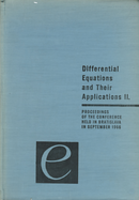## Differential Equations and Their Applications Proceedings of the Conference held in Bratislava in September 1966Editor: Šeda, Valter
Venue: Bratislava, 1966

Publisher: Slovenské pedagogické nakladateľstvo, Bratislava, 1967
Series: Acta Facultatis Rerum Naturalium Universitatis Comenianae. Mathematica, XVII

MR0249697
MSC: 34.00, 35.00

 5-6 Preface.
 7-8 Contents.

Comprehensive lectures presented in plenary sessions
 15-25 Problems of optimization of numerical mathematics.  Babuška, Ivo
 27-36 Algebraic elements in the transformation theory of 2nd order linear oscillatory differential equations.  Borůvka, O.
 37-43 On the convergence of difference schemes for classical and weak solutions of the Dirichlet problem.  Bramble, J. H.
 45-64 Nonlinear functional analysis and nonlinear partial differential equations.  Browder, E.
 65-72 Formally normal ordinary differential operators.  Coddington, E. A.
 73-80 Problems in linear control theory.  Conti, Roberto
 81-88 On linear differential equations of higher odd order.  Greguš, M.
 89-92 Invariant manifolds for flows.  Kurzweil, J.
 93-100 Vectors of Gevrey classes and applications.  Lions, J. L.
 101-119 On the existence and regularity of solutions of non-linear elliptic equations.  Nečas, J.
 121-127 Some boundary problems for the equations with strong degeneration.  Nikolskij, S.
 129-141 The $\Cal L^{p,\lambda}$ spaces and applications to the theory of partial differential equations.  Stampacchia, Guido
 143-153 Investigation of the solutions of differential equations on an infinite interval and the fixed point theorems.  Švec, Marko
 155-159 Arzela-like theorem with applications to differential equations and control theory.  Ważewski, T.

Comprehensive lectures presented in sections
 163-172 Axiomatization of differential equation theory.  Hájek, O.
 173-191 Invariant manifolds for discrete systems.  Halanay, A.
 193-199 On ordinary linear differential equations of high order.  Hornich, H.
 201-212 On the transformation of linear homogenous differential equations of the $n$-th order.  Hustý, Z.
 213-215 On bounded solutions of a certain differential equation.  Neuman, F.
 217-220 Asymptotic formulas for the solutions of the equation (py')' + qy = 0.  Ráb, M.
 221-235 An application of Green's function in the differential equations.  Šeda, V.
 237-242 Extension of the averaging method to stochastic equations.  Vrkoč, Ivo
 243-248 A general method of majorating of Dirichlet problem solutions.  Alexandrov, A. D.
 249-272 Structure of Green's operators and estimates for the corresponding eigenvalues.  Fichera, G.
 273-279 On the linear and quasilinear parabolic equations.  Ladyzenskaya, O. A.
 281-287 Some applications of the second method of Liapunov to dynamical systems described by partial differential equations.  Parks, P. C.
 289-292 Non-expansive mappings in convex linear topological spaces.  DePrima, C. R.
 293-300 Periodic solutions of nonlinear partial differential equations of evolution.  Vejvoda, O.
 301-305 Discretisation and error estimates for elliptic boundary value problems of the fourth order.  Zlámal, M.
 307-314 On some functions which verify differential inequalities.  Popoviciu, T.
 315-322 Stability of numerical processes.  Práger, M.; Vitásek, E.